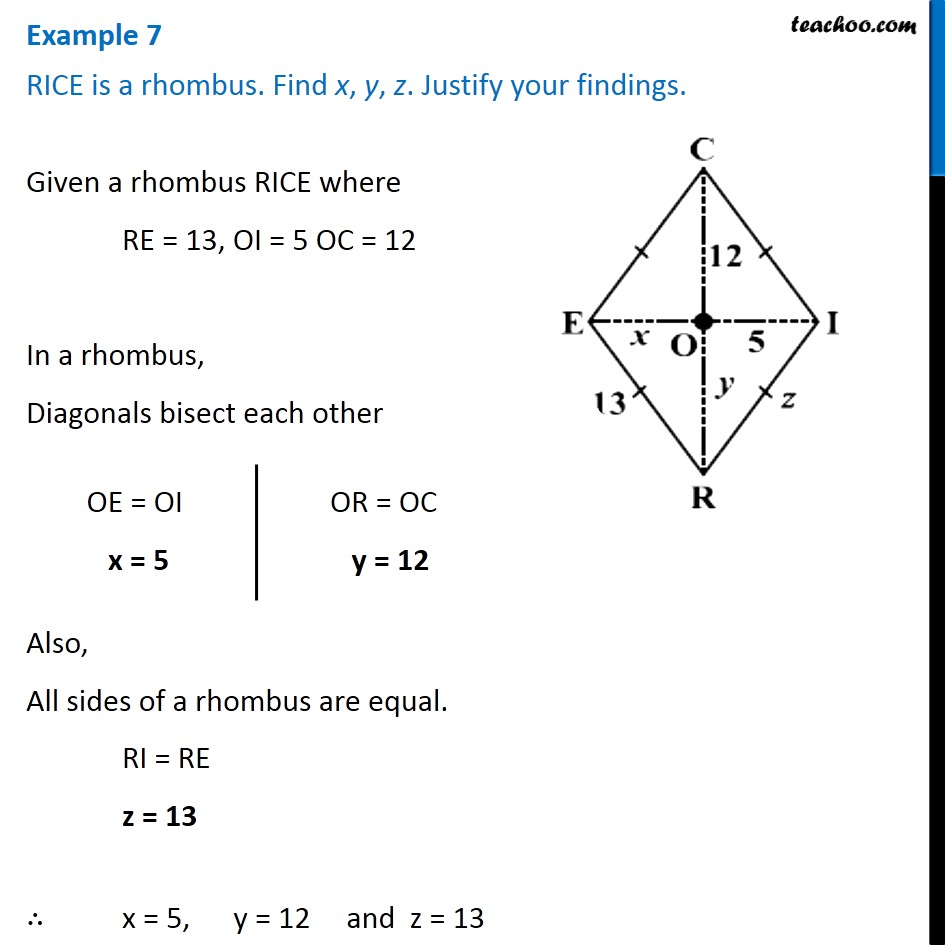Examples

Chapter 3 Class 8 Understanding Quadrilaterals
Serial order wise641 students have Teachoo Black. What are you waiting for?

### Transcript

Example 7 RICE is a rhombus. Find x, y, z. Justify your findings. Given a rhombus RICE where RE = 13, OI = 5 OC = 12 In a rhombus, Diagonals bisect each other OE = OI x = 5 OR = OC y = 12 Also, All sides of a rhombus are equal. RI = RE z = 13 ∴ x = 5, y = 12 and z = 13